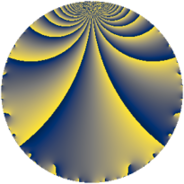# Properties

 Label 5520.2.a.boLevel $5520$ Weight $2$ Character orbit 5520.a Self dual yes Analytic conductor $44.077$ Analytic rank $0$ Dimension $2$ CM no Inner twists $1$

# Learn more about

## Newspace parameters

 Level: $$N$$ $$=$$ $$5520 = 2^{4} \cdot 3 \cdot 5 \cdot 23$$ Weight: $$k$$ $$=$$ $$2$$ Character orbit: $$[\chi]$$ $$=$$ 5520.a (trivial)

## Newform invariants

 Self dual: yes Analytic conductor: $$44.0774219157$$ Analytic rank: $$0$$ Dimension: $$2$$ Coefficient field: $$\Q(\sqrt{11})$$ Defining polynomial: $$x^{2} - 11$$ Coefficient ring: $$\Z[a_1, \ldots, a_{11}]$$ Coefficient ring index: $$1$$ Twist minimal: no (minimal twist has level 2760) Fricke sign: $$-1$$ Sato-Tate group: $\mathrm{SU}(2)$

## $q$-expansion

Coefficients of the $$q$$-expansion are expressed in terms of $$\beta = \sqrt{11}$$. We also show the integral $$q$$-expansion of the trace form.

 $$f(q)$$ $$=$$ $$q + q^{3} - q^{5} + 3 q^{7} + q^{9} +O(q^{10})$$ $$q + q^{3} - q^{5} + 3 q^{7} + q^{9} + ( 1 + \beta ) q^{11} + ( 1 - \beta ) q^{13} - q^{15} -\beta q^{17} + ( 5 - \beta ) q^{19} + 3 q^{21} - q^{23} + q^{25} + q^{27} + ( -4 + \beta ) q^{29} + ( 3 + 2 \beta ) q^{31} + ( 1 + \beta ) q^{33} -3 q^{35} + ( 5 + 2 \beta ) q^{37} + ( 1 - \beta ) q^{39} + \beta q^{41} + ( 4 - 2 \beta ) q^{43} - q^{45} + ( -1 - 3 \beta ) q^{47} + 2 q^{49} -\beta q^{51} -3 \beta q^{53} + ( -1 - \beta ) q^{55} + ( 5 - \beta ) q^{57} + ( 2 + 3 \beta ) q^{59} + ( 7 - \beta ) q^{61} + 3 q^{63} + ( -1 + \beta ) q^{65} + ( -9 + 2 \beta ) q^{67} - q^{69} + ( -2 + 3 \beta ) q^{71} + ( 5 - \beta ) q^{73} + q^{75} + ( 3 + 3 \beta ) q^{77} + q^{81} + ( -2 - \beta ) q^{83} + \beta q^{85} + ( -4 + \beta ) q^{87} + ( 2 + 2 \beta ) q^{89} + ( 3 - 3 \beta ) q^{91} + ( 3 + 2 \beta ) q^{93} + ( -5 + \beta ) q^{95} + 12 q^{97} + ( 1 + \beta ) q^{99} +O(q^{100})$$ $$\operatorname{Tr}(f)(q)$$ $$=$$ $$2q + 2q^{3} - 2q^{5} + 6q^{7} + 2q^{9} + O(q^{10})$$ $$2q + 2q^{3} - 2q^{5} + 6q^{7} + 2q^{9} + 2q^{11} + 2q^{13} - 2q^{15} + 10q^{19} + 6q^{21} - 2q^{23} + 2q^{25} + 2q^{27} - 8q^{29} + 6q^{31} + 2q^{33} - 6q^{35} + 10q^{37} + 2q^{39} + 8q^{43} - 2q^{45} - 2q^{47} + 4q^{49} - 2q^{55} + 10q^{57} + 4q^{59} + 14q^{61} + 6q^{63} - 2q^{65} - 18q^{67} - 2q^{69} - 4q^{71} + 10q^{73} + 2q^{75} + 6q^{77} + 2q^{81} - 4q^{83} - 8q^{87} + 4q^{89} + 6q^{91} + 6q^{93} - 10q^{95} + 24q^{97} + 2q^{99} + O(q^{100})$$

## Embeddings

For each embedding $$\iota_m$$ of the coefficient field, the values $$\iota_m(a_n)$$ are shown below.

For more information on an embedded modular form you can click on its label.

Label $$\iota_m(\nu)$$ $$a_{2}$$ $$a_{3}$$ $$a_{4}$$ $$a_{5}$$ $$a_{6}$$ $$a_{7}$$ $$a_{8}$$ $$a_{9}$$ $$a_{10}$$
1.1
 −3.31662 3.31662
0 1.00000 0 −1.00000 0 3.00000 0 1.00000 0
1.2 0 1.00000 0 −1.00000 0 3.00000 0 1.00000 0
 $$n$$: e.g. 2-40 or 990-1000 Significant digits: Format: Complex embeddings Normalized embeddings Satake parameters Satake angles

## Atkin-Lehner signs

$$p$$ Sign
$$2$$ $$1$$
$$3$$ $$-1$$
$$5$$ $$1$$
$$23$$ $$1$$

## Inner twists

This newform does not admit any (nontrivial) inner twists.

## Twists

By twisting character orbit
Char Parity Ord Mult Type Twist Min Dim
1.a even 1 1 trivial 5520.2.a.bo 2
4.b odd 2 1 2760.2.a.l 2
12.b even 2 1 8280.2.a.bc 2

By twisted newform orbit
Twist Min Dim Char Parity Ord Mult Type
2760.2.a.l 2 4.b odd 2 1
5520.2.a.bo 2 1.a even 1 1 trivial
8280.2.a.bc 2 12.b even 2 1

## Hecke kernels

This newform subspace can be constructed as the intersection of the kernels of the following linear operators acting on $$S_{2}^{\mathrm{new}}(\Gamma_0(5520))$$:

 $$T_{7} - 3$$ $$T_{11}^{2} - 2 T_{11} - 10$$ $$T_{13}^{2} - 2 T_{13} - 10$$ $$T_{17}^{2} - 11$$ $$T_{19}^{2} - 10 T_{19} + 14$$

## Hecke characteristic polynomials

$p$ $F_p(T)$
$2$ $$T^{2}$$
$3$ $$( -1 + T )^{2}$$
$5$ $$( 1 + T )^{2}$$
$7$ $$( -3 + T )^{2}$$
$11$ $$-10 - 2 T + T^{2}$$
$13$ $$-10 - 2 T + T^{2}$$
$17$ $$-11 + T^{2}$$
$19$ $$14 - 10 T + T^{2}$$
$23$ $$( 1 + T )^{2}$$
$29$ $$5 + 8 T + T^{2}$$
$31$ $$-35 - 6 T + T^{2}$$
$37$ $$-19 - 10 T + T^{2}$$
$41$ $$-11 + T^{2}$$
$43$ $$-28 - 8 T + T^{2}$$
$47$ $$-98 + 2 T + T^{2}$$
$53$ $$-99 + T^{2}$$
$59$ $$-95 - 4 T + T^{2}$$
$61$ $$38 - 14 T + T^{2}$$
$67$ $$37 + 18 T + T^{2}$$
$71$ $$-95 + 4 T + T^{2}$$
$73$ $$14 - 10 T + T^{2}$$
$79$ $$T^{2}$$
$83$ $$-7 + 4 T + T^{2}$$
$89$ $$-40 - 4 T + T^{2}$$
$97$ $$( -12 + T )^{2}$$
show more
show less